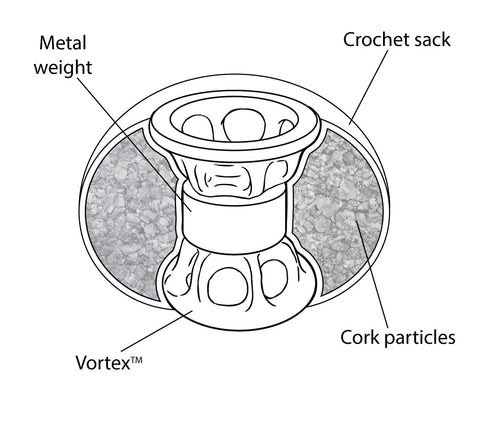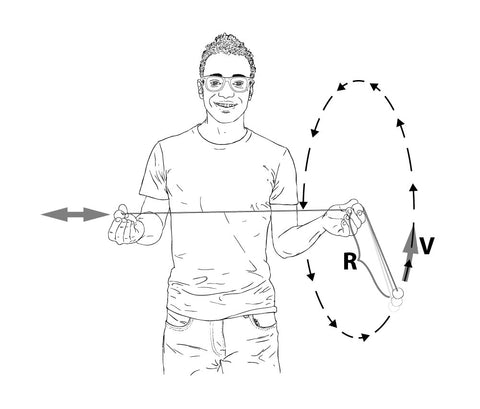# THE SCIENCE OF ASTROJAX

There's a lot of science in Astrojax's three balls on a string.  Astrojax has been used to teach physics at the grade school, high school, and college levels, NASA has taken Astrojax into outer space as part of its Toys in Space education program, and Astrojax has even been the subject of research on nonlinear dynamics and chaos using sophisticated computer modeling and advanced mathematics.  Here are a few examples of how this great educational toy demonstrates common scientific principles ...

## WHY IT'S FUN (SCIENTIFICALLY SPEAKING)

Astrojax walks the delicate balance between simplicity and complexity.  Three balls on a string is just simple enough to be controllable, yet just complicated enough to make things interesting.  Mathematically, things get complicated quite rapidly as you add "bodies."  For instance, two bodies alone in outer space orbit around each other in an ellipse.  It is easy to solve for that equation.  However, add just one more body and you get the "three body problem" ... and the mathematics can't be solved exactly, only approximately!  You can't get exact mathematical solutions again until you have many, many particles and you can analyze the system statistically.

Astrojax is fun to play with and seems to have a life force and mind of its own because it is chaotic.  Research on the system has determined the size and speed of the vertical orbit is infinitely sensitive to the size and speed of the tugs you give it.  You can't just hook the end ball up to a simple mechanical oscillator.  Your hand and brain have to continually monitor and correct for deviations in the orbits to keep it going. This chaotic aspect challenges you to explore, react, and be present and open to its whims and your own.

## WHY THE STRING DOESN'T TANGLEEach Astrojax ball has a metal weight in the center.  The metal weight at the center of the middle ball allows the middle ball to spin rapidly in response to the forces exerted by the string.  In science lingo we say that concentrating the mass at the center of an object lowers its "moment of inertia."  Divers use this principle of lowering their moment of inertia when they tuck into a ball during a dive to complete more rotations before they reach the water.  Similarly, ice skaters use this principle when they bring in their arms during a twirl to spin faster.

During vertical orbits, as the outer orbiting ball passes the string at the top of its orbit, the center ball has to rotate by 180 degrees in about 1/20th of a second in order for the string to not snag around the center ball.  The lowered moment of inertia produced by the central weighting, in combination with the shape of the bore, allows for this to happen.

## ORBITSWhen you perform horizontal orbits, the outer ball orbits about the center ball in a way similar to how the planets orbit around the sun, and how the moon orbits around the earth.  With Astrojax, the string attached to the outer orbiting ball produces a force on the outer orbiting ball which pulls in the direction towards the center of its circular path.  With the earth and the moon, the gravitation of the earth is the force which pulls in the direction towards the center of the moon's orbit to keep it circling around the earth.

## CONSERVATION OF ANGULAR MOMENTUM

If you hold an end ball in one hand and the middle ball in your other hand and spin the free end ball in circles, you will notice that as you pull the free end ball towards the middle ball it speeds up.Mathematically, what is happening is that the angular momentum, which is proportional to the product R x V (where R is the radius of the circle that the free end ball is orbiting in and V is the ball's velocity), is staying constant.  As the radius R decreases by, for instance, a factor of 2 as you pull the end ball in, the velocity V increases by a factor of 2.

Angular momentum is also conserved in the case of the ice skater mentioned above.  But in that case the calculation is more complicated because it is the *sum* of the product R x V for all the little points of mass in the body of the ice skater that stays constant.  As the points of mass in the ice skater's arms are brought closer in so that all their R's decrease, her rotational velocity V increases.

These explanations just scratch the surface of all the physics in Astrojax!